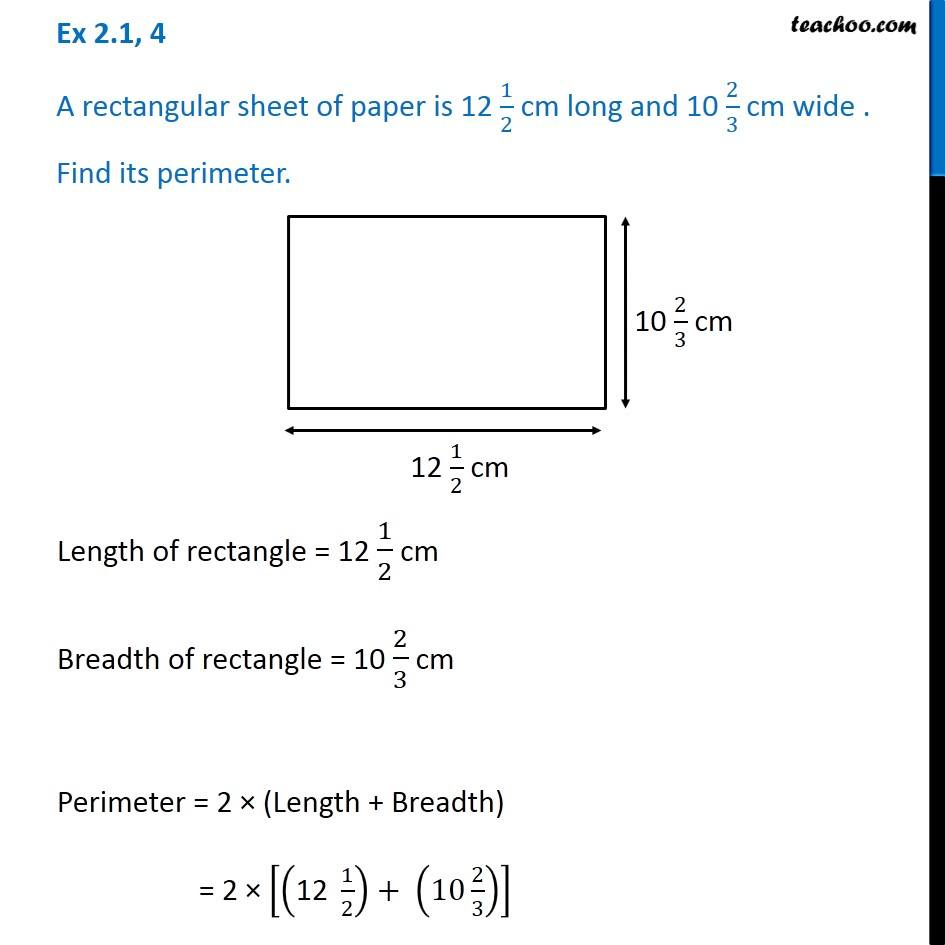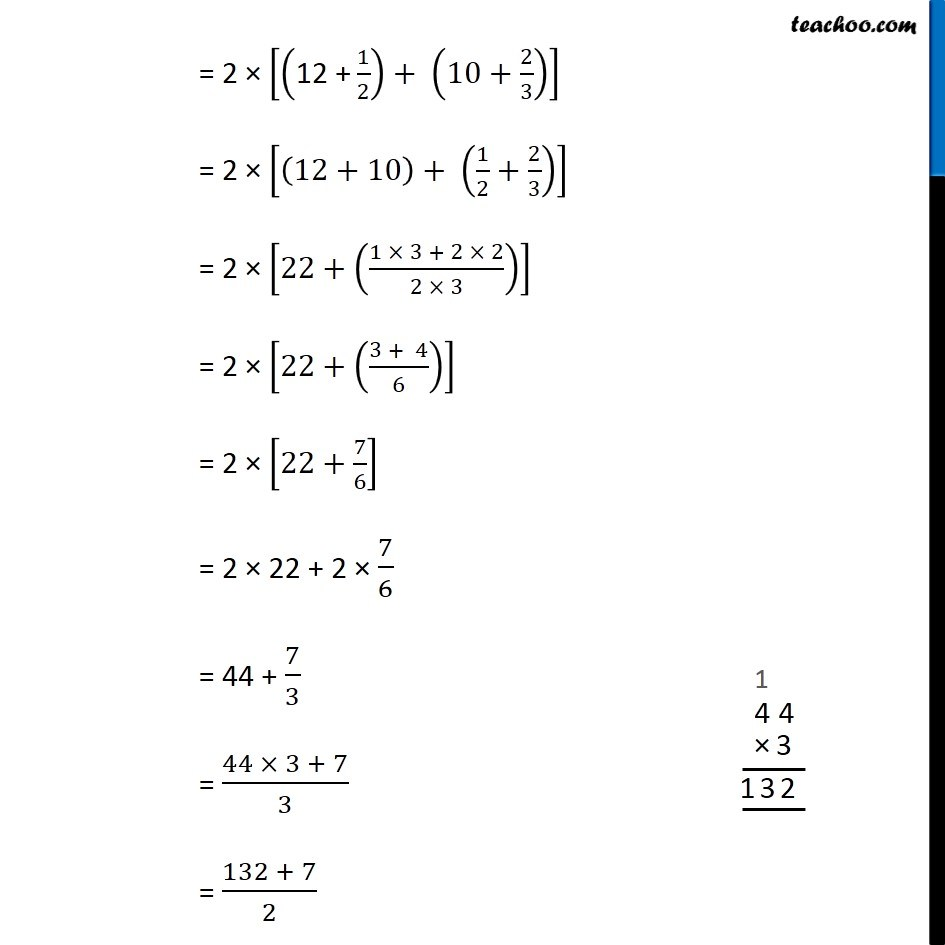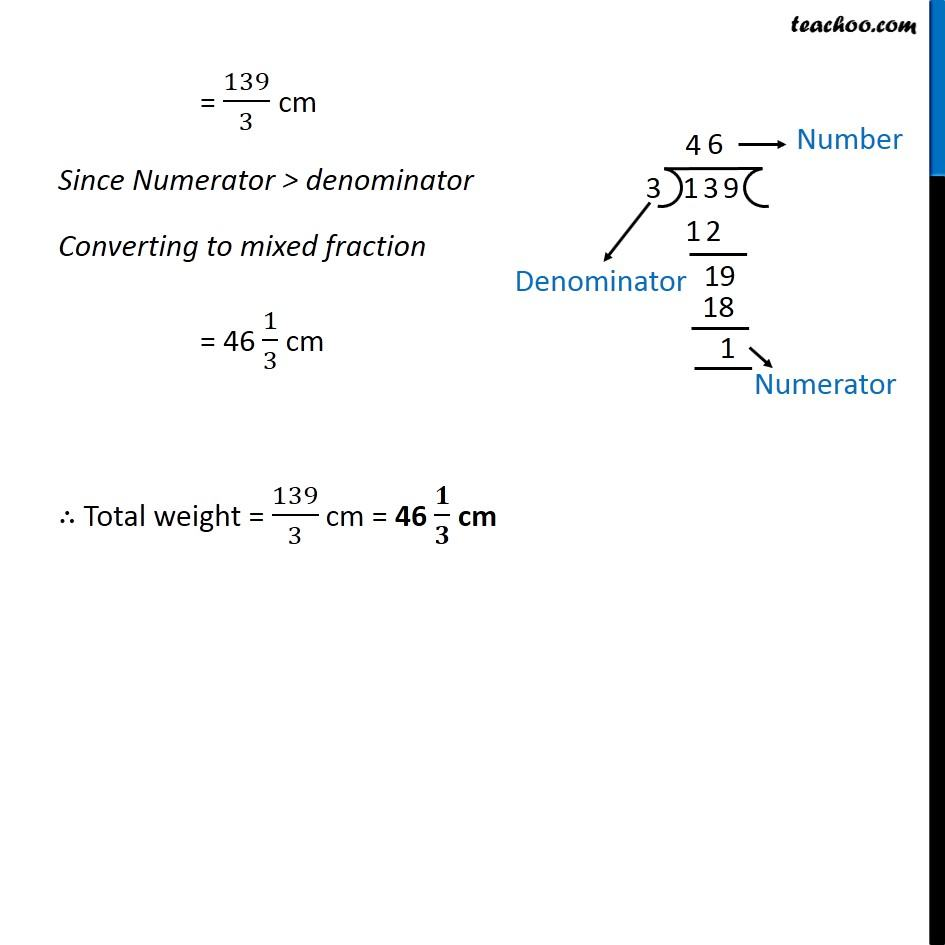1. Chapter 2 Class 7 Fractions and Decimals
2. Serial order wise
3. Ex 2.1

Transcript

Ex 2.1, 4 A rectangular sheet of paper is 12 1/2 cm long and 10 2/3 cm wide . Find its perimeter.Length of rectangle = 12 1/2 cm Breadth of rectangle = 10 2/3 cm Perimeter = 2 (Length + Breadth) = 2 [("12 " 1/2)+ (10 2/3)] = 2 [("12 +" 1/2)+ (10+2/3)] = 2 [(12+10)+ (1/2+2/3)] = 2 [22+((1 3 + 2 2)/(2 3))] = 2 [22+((3 + 4)/6)] = 2 [22+7/6] = 2 22 + 2 7/6 = 44 + 7/3 = (44 3 + 7)/3 = (132 + 7)/2 = 139/3 cm Since Numerator > denominator Converting to mixed fraction = 46 1/3 cm Total weight = 139/3 cm = 46 / cm

Ex 2.1

Chapter 2 Class 7 Fractions and Decimals
Serial order wise